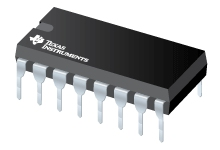CD4063B 4-Bit Magnitude Comparators | TI.com

# CD4063B (ACTIVE) 4-Bit Magnitude ComparatorsDatasheet

• Description
• Features
• Diagram
• Parametrics
• Complete your design

## Description

CD4063B is a 4-bit magnitude comparator designed for use in computer and logic applications that require the comparison of two 4-bit words. This logic circuit determines whether one 4-bit word (Binary or BCD) is "less than", "equal to", or "greater than" a second 4-bit word.

The CD4063B has eight comparing inputs (A3, B3, through A0, B0), three outputs (A < B, A = B, A > B) and three cascading inputs (A < B, A = B, A > B) that permit systems designers to expand the comparator function to 8, 12, 16 . . . 4N bits. When a single CD4063B is used, the cascading inputs are connected as follows: (A < B) = low, (A = B) = high, (A > B) = low.

For words longer than 4 bits, CD4063B devices may be cascaded by connecting the outputs of the less-significant comparator to the corresponding cascading inputs of the more-significant comparator. Cascading inputs (A < B, A = B, and A > B) on the least significant comparator are connected to a low, a high, and a low level, respectively.

The CD4063B types are supplied in 16-lead hermetic dual-in-line ceramic packages (F3A suffix), 16-lead dual-in-line plastic packages (E suffix), 16-lead small-outline packages (M, M96, MT and NSR suffixes), and 16-lead thin shrink small-outline packages (PW and PWR suffixes). This device is pin-compatible with the standard 7485 TTL type.

## Features

• Expansion to 8, 12, 16...4N bits by cascading units
• Medium-speed operation:
compares two 4-bit words in 250 ns (typ.) at 10 V
• 100% tested for quiescent current at 20 V
• Standardized symmetrical output characteristics
• 5-V, 10-V, and 15-V parametric ratings
• Maximum input current of 1 µA at 18 V over full package temperature range; 100 nA at 18 V and 25°C
• Noise margin (full package temperature range) =
• 1 V at VDD = 5 V
• 2 V at VDD = 10 V
• 2.5 V at VDD = 15 V
• Meets all requirements of JEDEC Tentative Standard No. 13B, "Standard Specifications for Description of ’B’ Series CMOS Devices"
• Applications
• Servo motor controls
• Process controllers

Data sheet acquired from Harris Semiconductor.